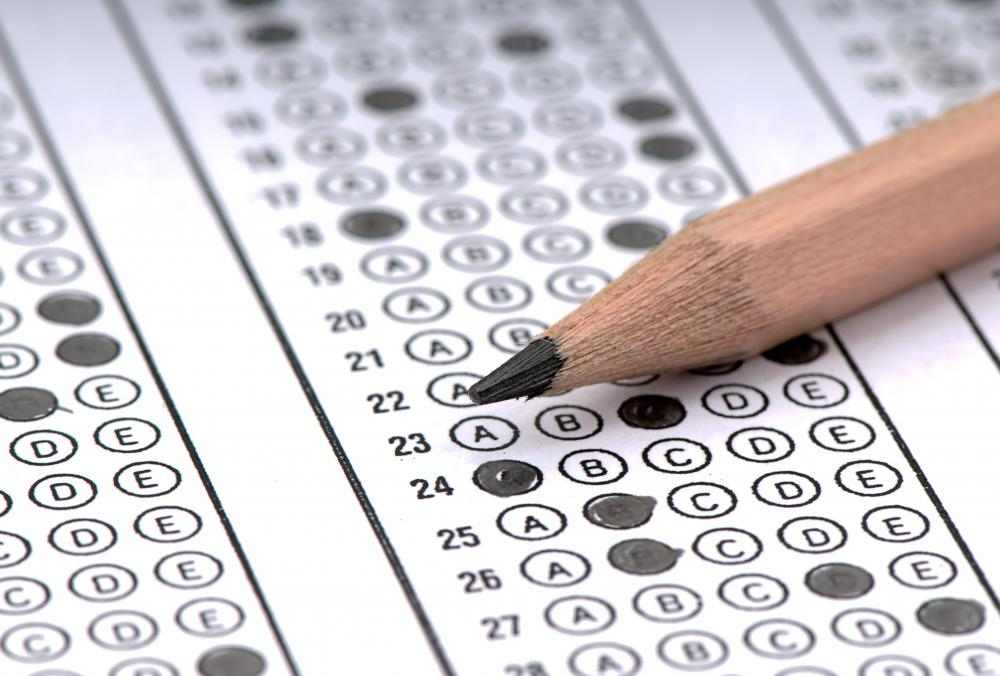# How Do I Score Well on Praxis I® Math? (with pictures)

Gabriele Sturmer
Gabriele SturmerThe Praxis I Math Exam has four sections.

The Praxis I® math, or Praxis I Pre-Professional Skills Test® in Mathematics, is one of the Educational Testing Service's American teacher certification exams. It includes four sections of multiple-choice questions that cover topics ranging from basic mathematical operations to algebra and probability. The exam requires that you have a basic knowledge of mathematics, so you'll need to review and practice topics in each of the exam's sections to score well on the Praxis I® math.Praxis I math exam questions are multiple choice.

The first section, Number and Operations, covers number properties and operations, place value, estimation, ratios and numerical reasoning. The Praxis I® math does not allow the use of a calculator, so you'll need to feel comfortable adding, subtracting, multiplying and dividing manually. In addition, you should feel comfortable solving word problems that require ratios and percentages. The best way to practice for this section is to download worksheets online, purchase a workbook on basic mathematics, or both.

Algebra makes up the second section of the Praxis I® math exam and requires you to solve equations, identify patterns, solve word problems and use deductive reasoning. To best prepare for the algebra section, you should review how to create equations and how to solve for unknown values. Purchasing a college or high school algebra textbook is a good way to prepare, especially if the book has practice problems and answers in the index. Using flash cards for learning the formulas is also helpful.

The third section covers topics in geometry and measurement, such as using geometric formulas and converting to and from the linear and metric systems. You'll be asked to identify congruence in shapes and to use the Pythagorean theorem to solve triangles. You'll also need to be familiar with coordinate geometry and feel comfortable locating and solving problems with given coordinates on a plane. While studying a geometry textbook and solving problems is helpful for the first portion of this section, you may find it more useful to use flashcards for learning measurement conversions.

Data analysis and probability topics fill the final portion of the Praxis I® math. You'll need to know how to interpret information from graphs and understand data sets gathered from the graphs. You should learn how to find the mean, median, range and mode for a data set and also how to determine the probability of an outcome. Online guides or textbooks are useful for learning these topics.

## You might also Like## Discuss this Article

Forgot password?
###### Register:
•The Praxis I Math Exam has four sections.
•Praxis I math exam questions are multiple choice.